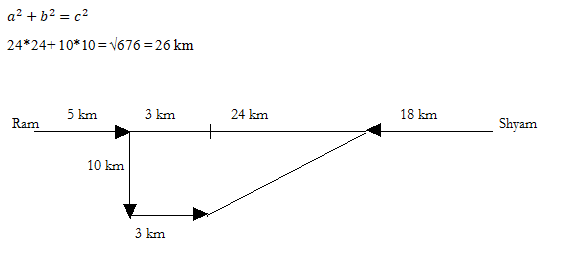# SSC CGL 2018 Practice Test Papers | Reasoning Ability (Day-6)

Dear Aspirants, Here we have given the Important SSC CGL Exam 2018 Practice Test Papers. Candidates those who are preparing for SSC CGL 2018 can practice these questions to get more confidence to Crack SSC CGL 2018 Examination.

[WpProQuiz 2688]

Click “Start Quiz” to attend these Questions and view Explanation

1. Which answer figure will complete the pattern in the question figure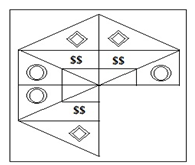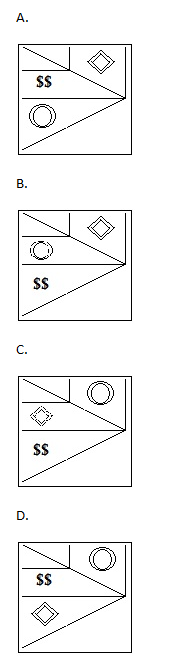1. From the given answer select in one in which the question figure is hidden / embedded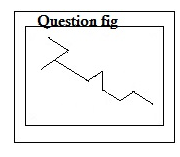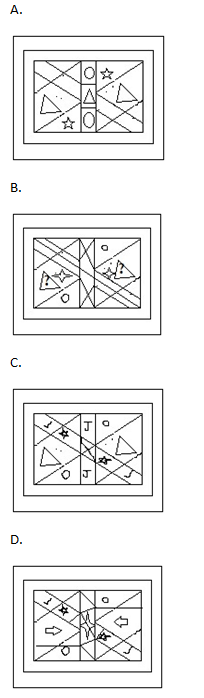1. A piece of paper is folded and punched as shown below in the question figures. From the given answer figures, indicate how it will appear when opened.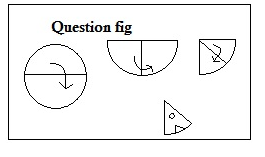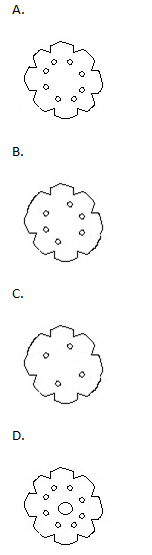1. A word is represented by only one set of numbers as given in any one of the alternatives. The sets of numbers given in the alternatives are represented by two classes of alphabets as shown in the given two matrices. The columns and rows of Matrix-I are numbered from 0 to 4 and that of Matrix-II are numbered from 5 to 9. A letter from these matrices can be represented first by its row and next by its column, for example, ‘E’ can be represented by 01,78, etc., and ‘I’ can be represented by 02, 13, etc. similarly, you have to identify the set for the word

“HAMLET”.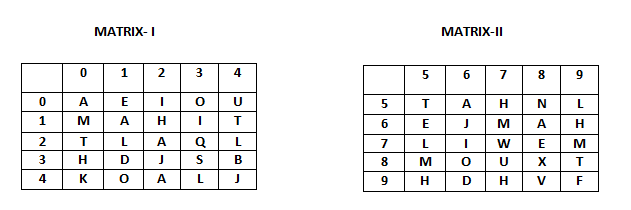1. 57, 00, 21, 11, 01, 20
2. 30, 68, 67, 43, 69, 14
3. 12, 68, 33, 75, 41, 55
4. 95, 22, 79, 21, 78, 89
1. Find out the wrong number in the series

22 36 60 95 114

A.22

B.36

C.95

D.60

1. What will come in place of question mark?

14 5 94 ? 219 316

A.156

B.161

C.118

D.178

1. If ‘REPLACE’ is written as ‘EDCOMDR’, then ‘SERVICE’ should be written as

A.EDLYPOS

B.SDKTPUC

C.EDKYPDS

D.DDMWJA

1. Statement: Some fruits are flowers. No flower is leaf. All trees are leaf.

Conclusion:

I.Some fruits are trees.

II.Some leaves are fruits.

III. No leaf is a fruit

A.Only  I follows

B.Only II follows

C.None follows

D.Either II or III follows

1. In the English alphabet series if all vowels were dropped then which of the letter will be the 8th from the right of 7th from the left end?

A.S

B.J

C.T

D.R

1. Ram and Shyam are 60kms apart from each other, they start walking towards each other. After 5km Ram turned right and walk for 10km before turning left, he then covered a distance of 3km. If both are moving with same speed, find the shortest distance between them.

A.30√2

B.22√3

C.26

D.24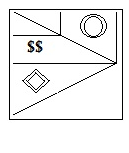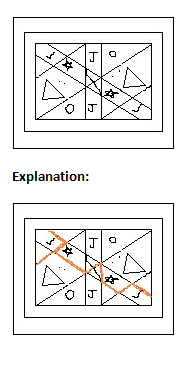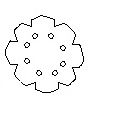95, 22, 79, 21, 78, 89

4*1+5*1=9

5*2+6*2=22

6*3+7*3=39

7*4+8*4=60

8*5+9*5=95

9*6+10*6=114

2*2+10=14

5*5+20=45

8*8+30=94

11*11+40=161

13*13+50=219

16*16+60=256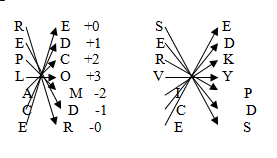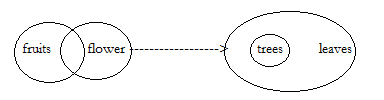Letter A B C D E F G H I J K L M Position 1 2 3 4 5 6 7 8 9 10 11 12 13
 Letter N O P Q R S T U V W X Y Z Position 14 15 16 17 18 19 20 21 22 23 24 25 26